Chapter 6 Part 1 - Foreign Exchange Rates

Economics Class 12
Macroeconomics

##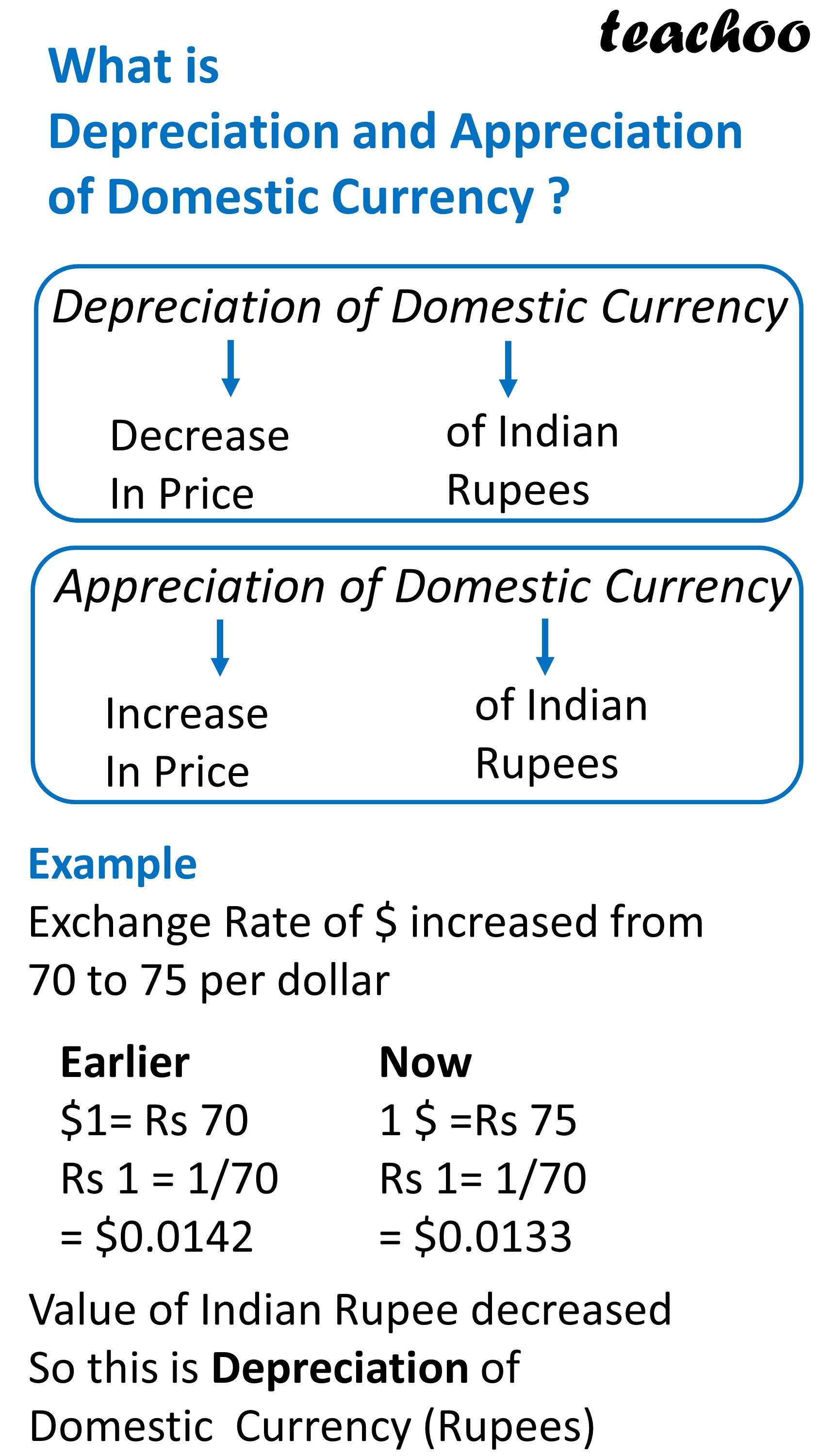googletag.cmd.push(function() { googletag.display('div-gpt-ad-1669298377854-0'); });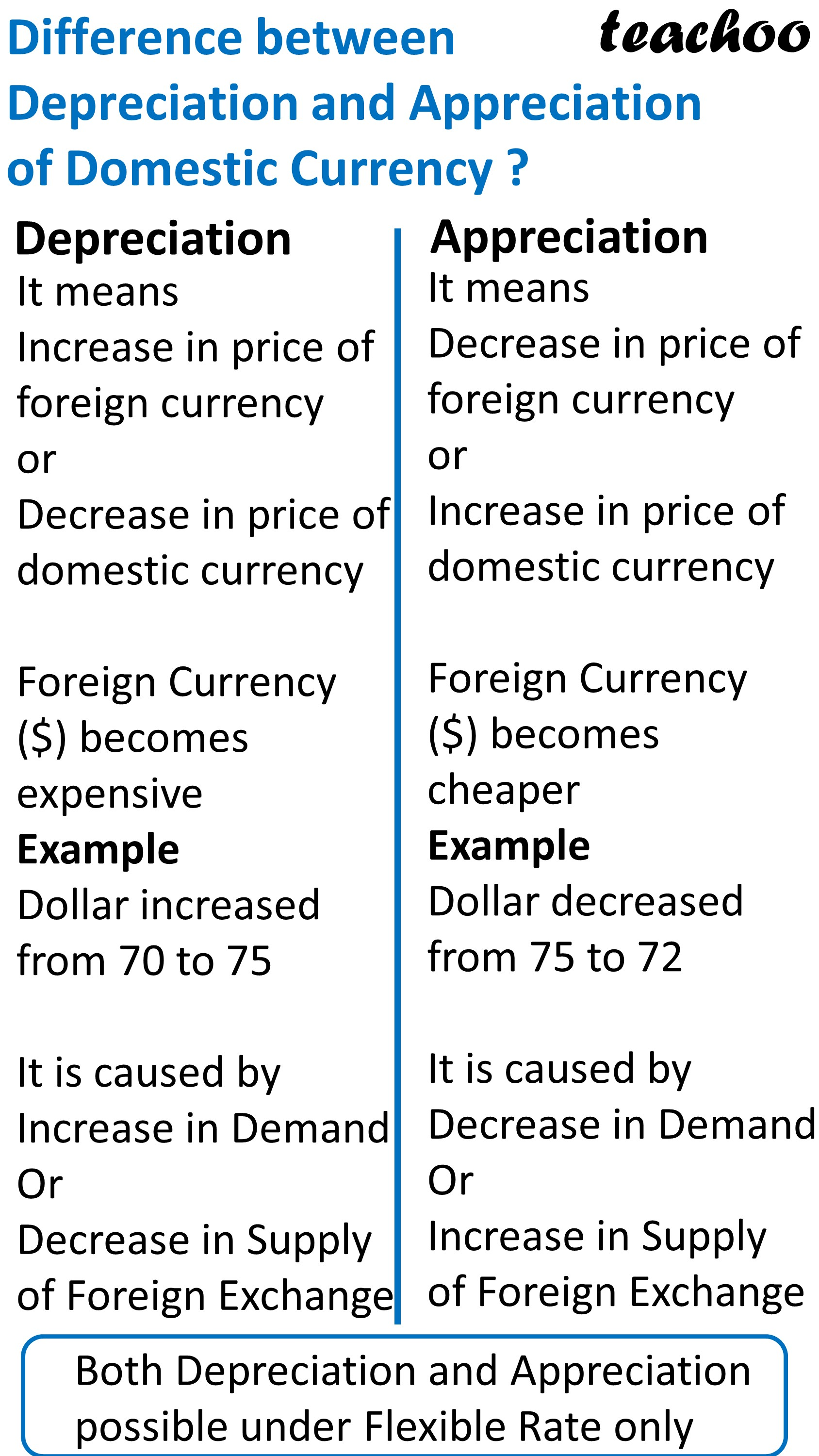## What causes Depreciation of Domestic Currency?

It means Increase in price of Foreign Currency as compared to indian Currency

Example

Suppose Exchange Rate is Rs 70

After 1 year, it Increases to Rs 75

It means Value of Dollar has increased as compared to Indian Rupee

We can also say that, Value of Indian Rupees has depreciated (decreased) as compared to US Dollar

## Reason for Depreciation of Domestic Currency

It is caused by Increase in Demand of Foreign Exchange or Decrease in Supply of Foreign Exchange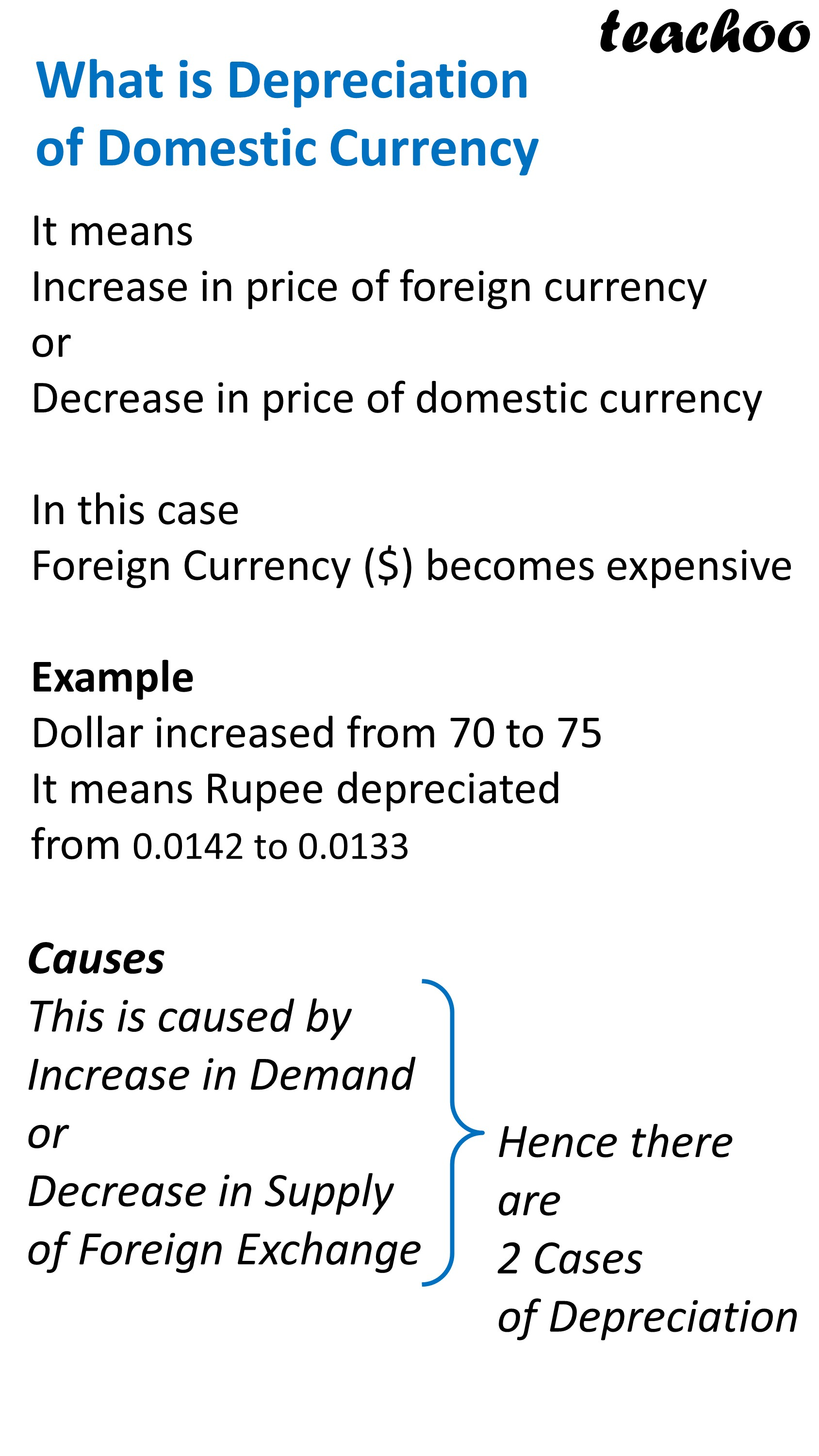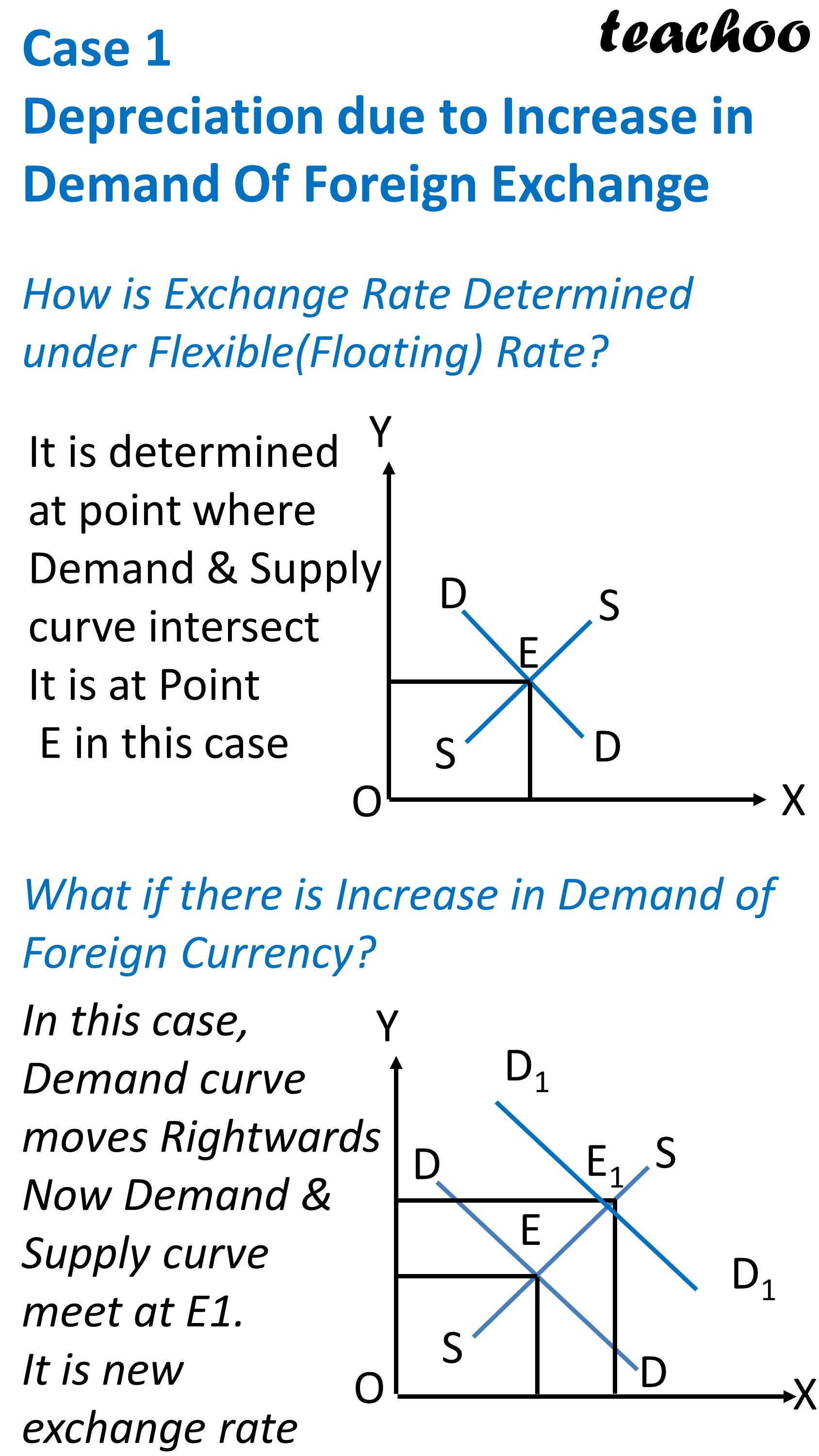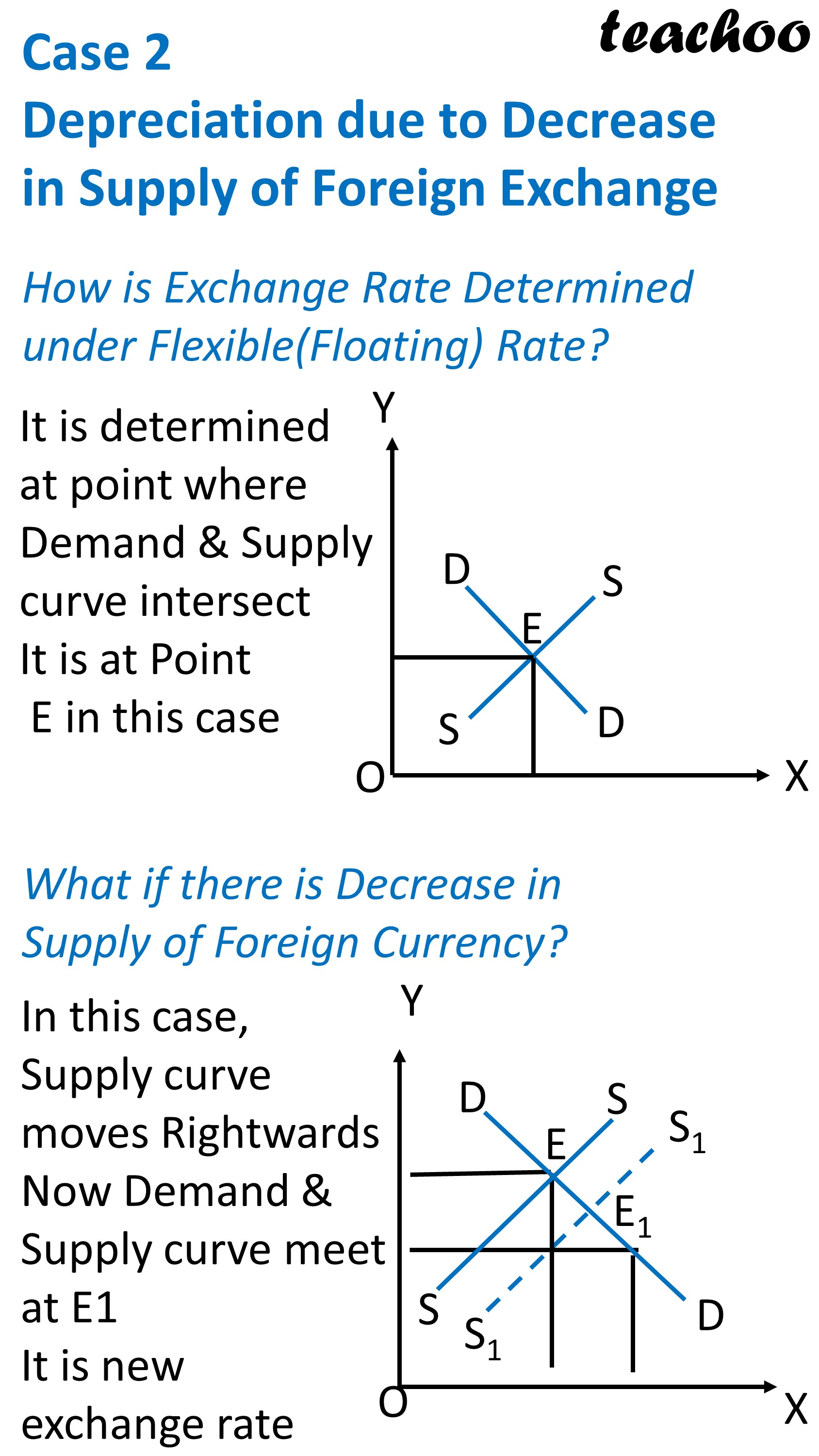## What causes Appreciation of Domestic Currency?

It means decrease in price of Foreign Currency as compared to Indian Currency

It is caused by Increase in Supply of Foreign Exchange

Example

Suppose Exchange Rate is Rs 70

After 1 year, it Decreases to Rs 65

It means Value of Dollar has decreased as compared to Indian Rupee

We can also say that, Value of Indian Rupees has appreciated (increased) as compared to US Dollar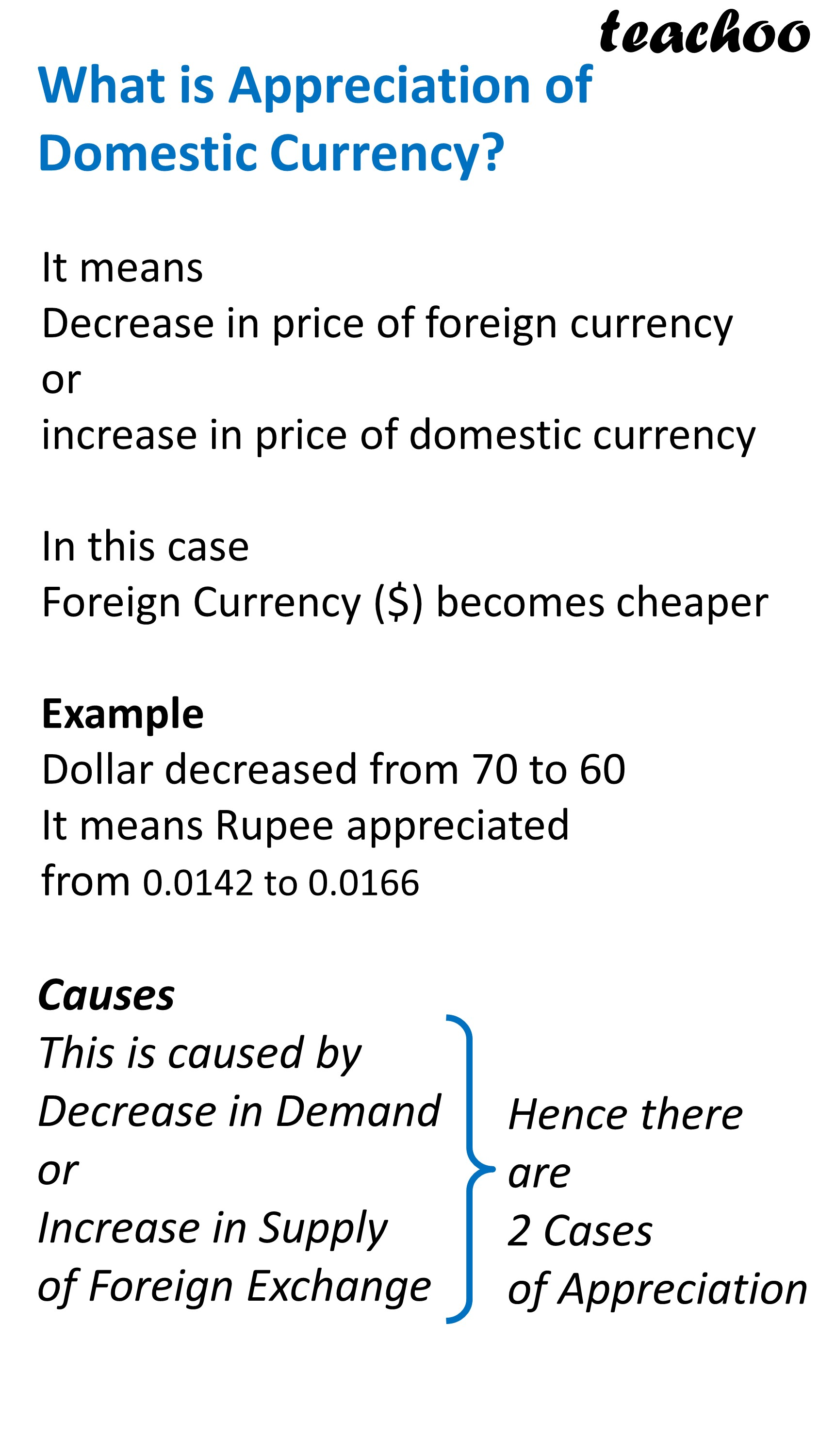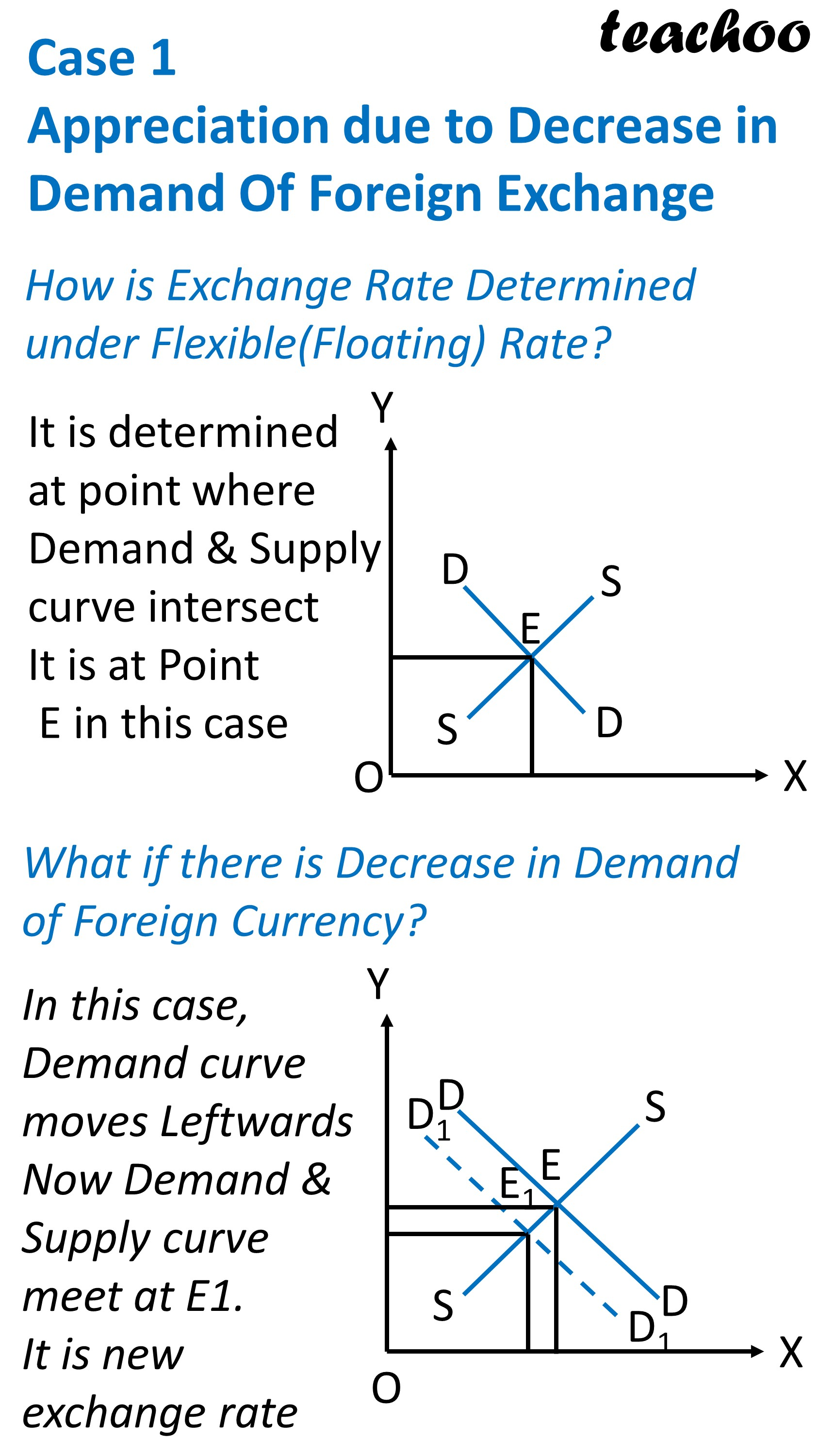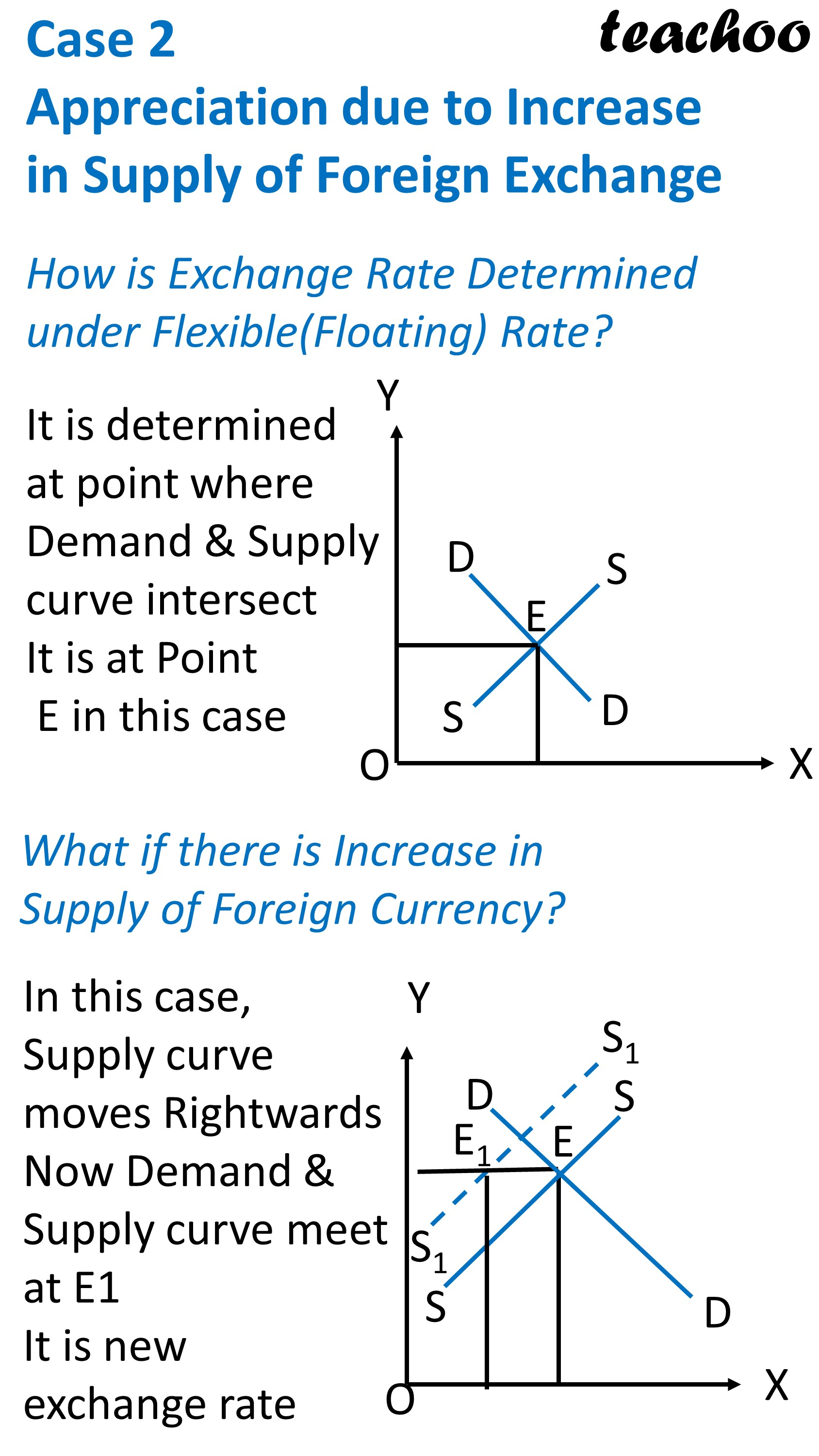### NCERT Questions

No questions in this part

### Other Books

#### Question 1

In the following questions, select the correct answers:

A change from Rs 140 = \$2 to Rs 60 = \$1 indicates that Rs is:

1. Appreciating
2. Depreciating
3. Neither A nor B
4. Either A or B

#### Question 2

Depreciation of domestic currency leads to rise in:

1. Exports
2. Imports
3. Either A or B
4. Neither A nor B

#### Question 3

The value of US Dollar \$1 has gone down from Rs73 to Rs70.

This will lead to rise in:

1. Exports to USA
2. Imports from USA
3. Both A and B
4. Either A or B

Learn in your speed, with individual attention - Teachoo Maths 1-on-1 Class

### Transcript

What is Depreciation and Appreciation of Domestic Currency ? Depreciation of Domestic Currency Decrease In Price of Indian Rupees Appreciation of Domestic Currency Increase In Price of Indian Rupees Example Exchange Rate of \$ increased from 70 to 75 per dollar Earlier \$1= Rs 70 Rs 1 = 1/70 = \$0.0142 Now 1 \$ =Rs 75 Rs 1= 1/70 = \$0.0133 Value of Indian Rupee decreased So this is Depreciation of Domestic Currency (Rupees) Difference between Depreciation and Appreciation of Domestic Currency ? Depreciation It means Increase in price of foreign currency or Decrease in price of domestic currency Foreign Currency (\$) becomes expensive Example Dollar increased from 70 to 75 It is caused by Increase in Demand Or Decrease in Supply of Foreign Exchange Appreciation It means Decrease in price of foreign currency or Increase in price of domestic currency Foreign Currency (\$) becomes cheaper Example Dollar decreased from 75 to 72 It is caused by Decrease in Demand Or Increase in Supply of Foreign Exchange Both Depreciation and Appreciation possible under Flexible Rate only What is Depreciation of Domestic Currency It means Increase in price of foreign currency or Decrease in price of domestic currency In this case Foreign Currency (\$) becomes expensive Example Dollar increased from 70 to 75 It means Rupee depreciated from 0.0142 to 0.0133 Causes This is caused by Increase in Demand or Decrease in Supply of Foreign Exchange Hence there are 2 Cases of Depreciation Case 1 Depreciation due to Increase in Demand Of Foreign Exchange How is Exchange Rate Determined under Flexible(Floating) Rate? It is determined at point where Demand & Supply curve intersect It is at Point E in this case What if there is Increase in Demand of Foreign Currency? In this case, Demand curve moves Rightwards Now Demand & Supply curve meet at E1. It is new exchange rate Case 2 Depreciation due to Decrease in Supply of Foreign Exchange How is Exchange Rate Determined under Flexible(Floating) Rate? It is determined at point where Demand & Supply curve intersect It is at Point E in this case What if there is Decrease in Supply of Foreign Currency? In this case, Supply curve moves Rightwards Now Demand & Supply curve meet at E1 It is new exchange rate What is Appreciation of Domestic Currency? It means Decrease in price of foreign currency or increase in price of domestic currency In this case Foreign Currency (\$) becomes cheaper Example Dollar decreased from 70 to 60 It means Rupee appreciated from 0.0142 to 0.0166 Causes This is caused by Decrease in Demand or Increase in Supply of Foreign Exchange Hence there are 2 Cases of Appreciation Case 1 Appreciation due to Decrease in Demand Of Foreign Exchange How is Exchange Rate Determined under Flexible(Floating) Rate? It is determined at point where Demand & Supply curve intersect It is at Point E in this case What if there is Decrease in Demand of Foreign Currency? In this case, Demand curve moves Leftwards Now Demand & Supply curve meet at E1. It is new exchange rate Case 2 Appreciation due to Increase in Supply of Foreign Exchange How is Exchange Rate Determined under Flexible(Floating) Rate? It is determined at point where Demand & Supply curve intersect It is at Point E in this case What if there is Increase in Supply of Foreign Currency? In this case, Supply curve moves Rightwards Now Demand & Supply curve meet at E1 It is new exchange rate